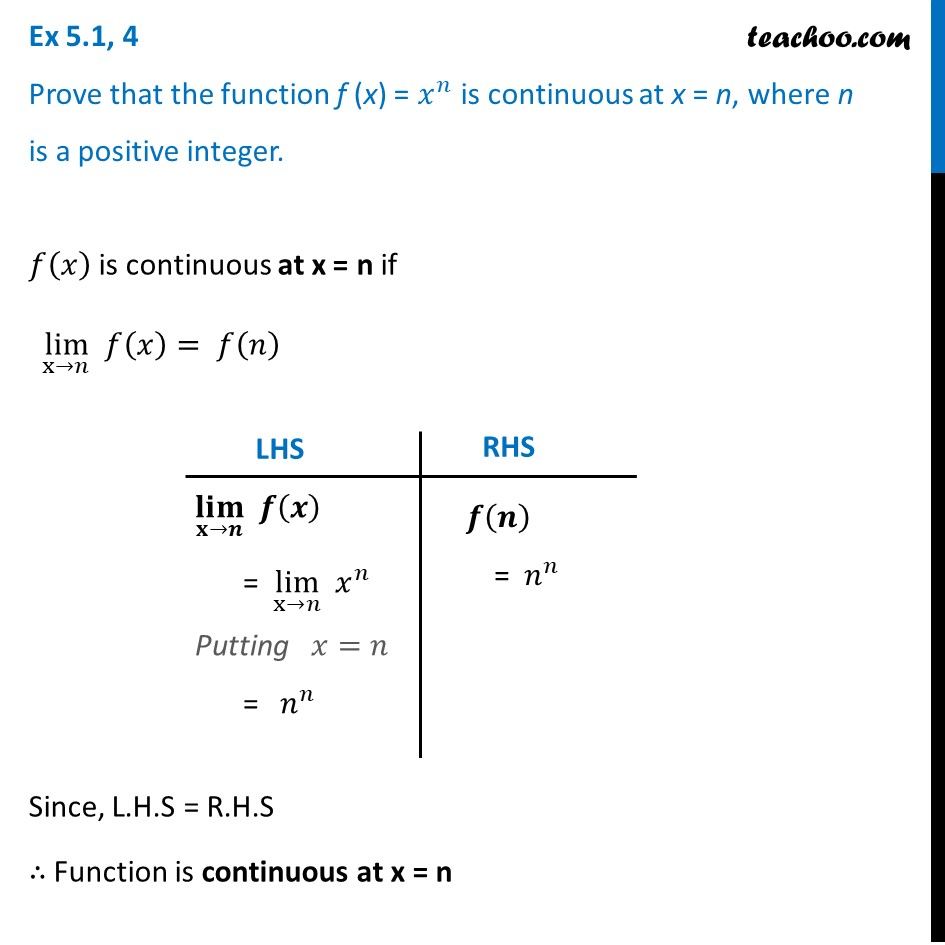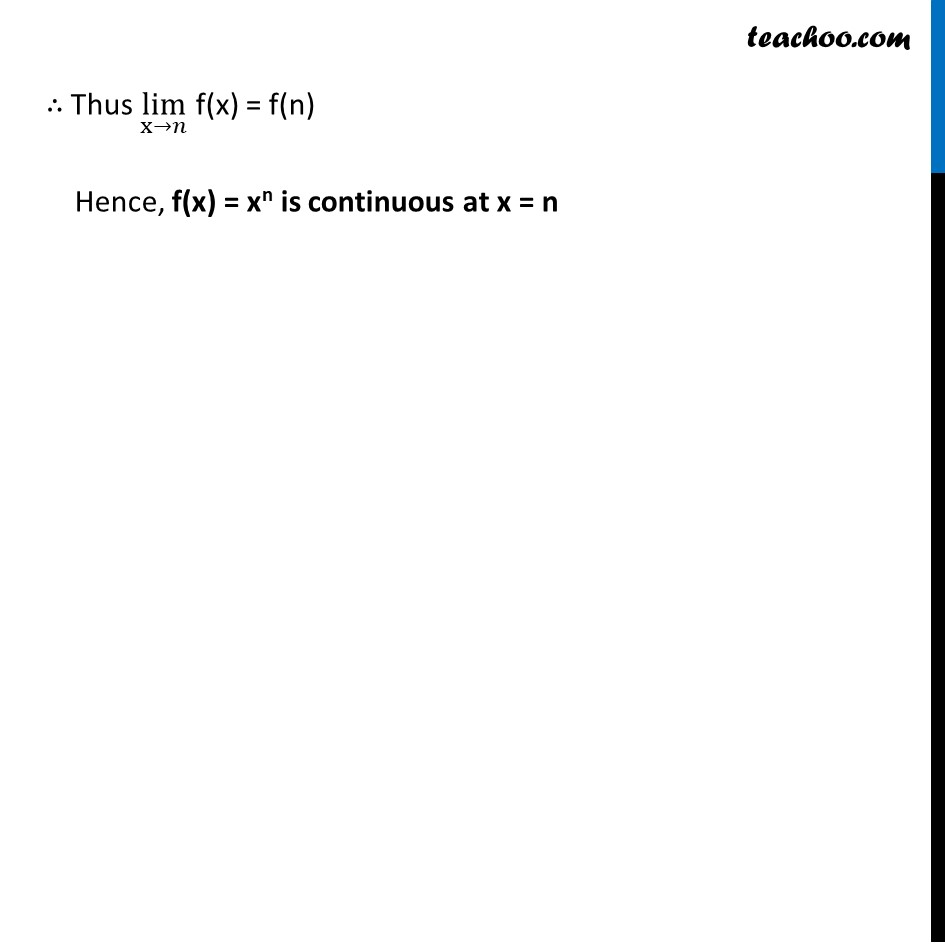1. Chapter 5 Class 12 Continuity and Differentiability (Term 1)
2. Serial order wise
3. Ex 5.1

Transcript

Ex 5.1, 4 Prove that the function f (x) = 𝑥^𝑛 is continuous at x = n, where n is a positive integer.𝑓(𝑥) is continuous at x = n if lim┬(x→𝑛) 𝑓(𝑥)= 𝑓(𝑛) Since, L.H.S = R.H.S ∴ Function is continuous at x = n (𝐥𝐢𝐦)┬(𝐱→𝒏) 𝒇(𝒙) = lim┬(x→𝑛) 𝑥^𝑛 Putting 𝑥=𝑛 = 𝑛^𝑛 𝒇(𝒏) = 𝑛^𝑛 ∴ Thus lim┬(x→𝑛) f(x) = f(n) Hence, f(x) = xn is continuous at x = n